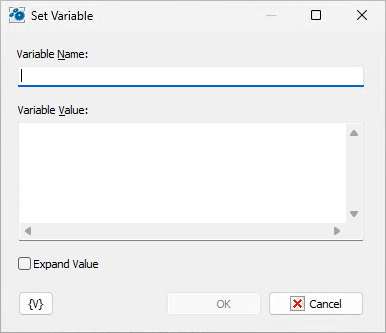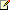﻿ Set Variable - RoboTask User's Guide
Keyword Index

 Set Variable

Defines the value of a variable when you run a task.Variable Name

Enter the name of the variable you want to set the value of.

Variable Value

Enter the value that you want to set for the variable.

Expand variable value.

If this parameter is on, RoboTask computes the value of an expression (that is, expands variables in the expression) before assigning a value to the specified variable.

Otherwise, the value is assigned without expanding variables. In this case the value is computed while further using the variable. Meanwhile, the variable value and the value of all variables are expanded recursively until the very end.

There can be necessity of using both modes in tasks:

 • Expand variable value is on – when you have to fix the value in the moment of assigning it to the variable. For example: current time
 • Expand variable value is off – when you have to compose a complex expression of many variables and then you have to use only one variable in further steps. You also don’t need to turn on the expanding of variables if an assigned expression contains a static text and doesn’t contain other variables.

By default, the parameter is off.Related Topics

Create Variable

Remove Variable

Increment Variable

Delimited variable

TimeDifference